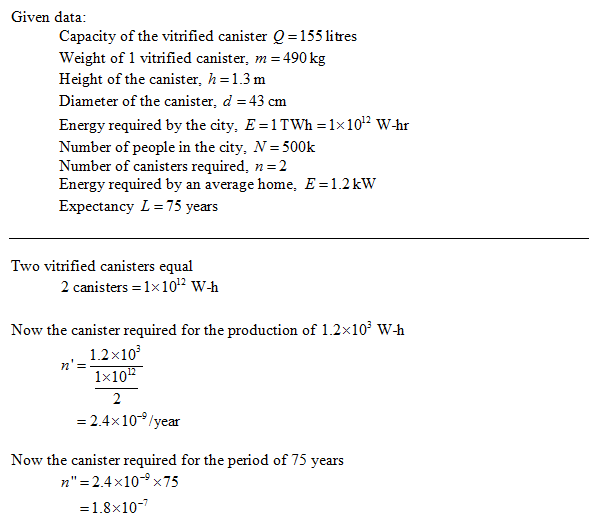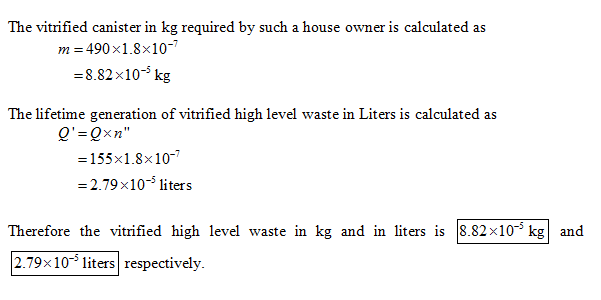In: Physics

# Given :Vitrified canister equal 1.5 fuel assemblies (f.p. and MA; Capacity: 155 litres; weight: 490 kg;...

Given :Vitrified canister equal 1.5 fuel assemblies (f.p. and MA; Capacity: 155 litres; weight: 490 kg; height: 1.3 m; diameter: 43 cm). An amount ofabout 1 TWh enough for a city of 500k for year takes two canisters.

Assume the average home requires an electrical power of 1.2kW, an average life expectancy of 75 years, and only power from nuclear energy. For such a homeowner, what would be the lifetime generation of vitrified high level waste (HLW) in kg and liters?

## Solutions

##### Expert Solution## Related Solutions

##### Particle A (mA = 1 kg) is given initial velocity 10 m/s to the right, while...
Particle A (mA = 1 kg) is given initial velocity 10 m/s to the right, while particle B (mB = 1:5 kg) is initially at rest. The coecient of restitution for any impact between A and B is 2/3 while the coecient of restitution for impact of either particle with either wall is 5/6. The particles slide along a smooth horizontal supporting surface. Compute the percentage of the kinetic energy remaining in the system of A and B at the...
##### Two grapes are given equal charges and held apart at a distance of 1.5 m. They...
Two grapes are given equal charges and held apart at a distance of 1.5 m. They experience a repulsive force of 2.2 N. Find the magnitude of the charge on each grape.
##### An elevator has a weight capacity of 3000 lbs. Sixteen men get into the elevator. Given...
An elevator has a weight capacity of 3000 lbs. Sixteen men get into the elevator. Given the weights of men are normally distributed with a mean of 172 lbs and a std dev of 29 lbs find the probability of these men exceeding the maximum weight, thus breaking the cable and plunging to their death. _________
##### An intramuscular medication is given at 3.00mg per kg body weight. If the patient weighs 157...
An intramuscular medication is given at 3.00mg per kg body weight. If the patient weighs 157 pounds, how much medication is needed? Show work